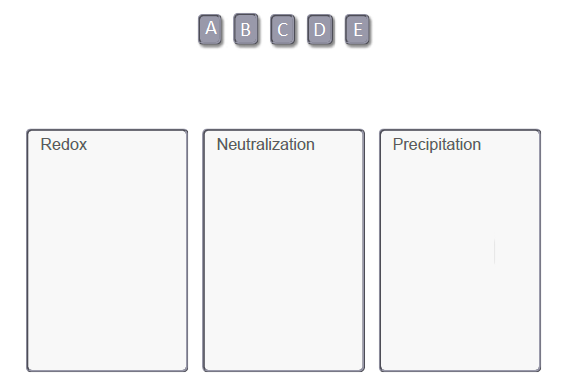# Problem: Part A. Classify the following reactions:A. HNO3(aq) + NH3(aq) → NH4NO3(aq) + H2O(l)B. 2 Cu(s) + O2(g) → 2 CuO(s)C. NaCl(aq) + AgNO3(aq) → NaNO3(aq) + AgCl(s)D. 2 LiOH(aq) + H2SO4(aq) → Li2SO4(aq) + 2 H2O(l)E. CaCl2(aq) + K2CO3(aq) → 2 KCl(aq) + CaCO3(s)Drag the items to their respective bins.Reactions in water solution can be classified based on the driving force that converts reactants to product. The three classes are precipitation reactions, acid-base neutralization reactions, and oxidation-reduction reactions.• In precipitation reactions a solid product forms from aqueous reactants. The product can be predicted by using solubility rules.• In acid-base neutralization reactions an acid (proton donor) reacts with a base (proton acceptor) to form an ionic salt and sometimes water. You can recognize this type of reaction by the presence of an acid and base in the reactants.• In oxidation-reduction reactions (or redox for short) electrons are transferred between reactants. So you will always see changes in oxidation number within this type of reaction.

###### FREE Expert SolutionView Complete Written Solution
###### Problem Details

Part A. Classify the following reactions:

A. HNO3(aq) + NH3(aq) → NH4NO3(aq) + H2O(l)
B. 2 Cu(s) + O2(g) → 2 CuO(s)
C. NaCl(aq) + AgNO3(aq) → NaNO3(aq) + AgCl(s)
D. 2 LiOH(aq) + H2SO4(aq) → Li2SO4(aq) + 2 H2O(l)
E. CaCl2(aq) + K2CO3(aq) → 2 KCl(aq) + CaCO3(s)

Drag the items to their respective bins.Reactions in water solution can be classified based on the driving force that converts reactants to product. The three classes are precipitation reactions, acid-base neutralization reactions, and oxidation-reduction reactions.

• In precipitation reactions a solid product forms from aqueous reactants. The product can be predicted by using solubility rules.
• In acid-base neutralization reactions an acid (proton donor) reacts with a base (proton acceptor) to form an ionic salt and sometimes water. You can recognize this type of reaction by the presence of an acid and base in the reactants.
• In oxidation-reduction reactions (or redox for short) electrons are transferred between reactants. So you will always see changes in oxidation number within this type of reaction.